# Feasibility Using Problem-Based `Optimize` Live Editor Task

### Problem Description

This example shows how to find a feasible point using the `Optimize` Live Editor task using a variety of solvers. The problem is to find a point $\left[x,y\right]$ satisfying these constraints:

`$\left(y+{x}^{2}{\right)}^{2}+0.1{y}^{2}\le 1$`

`$y\le \mathrm{exp}\left(-x\right)-3$`

$y\le x-4$.

Graph the curves where the constraint functions are equal to zero. To see which part of the region is feasible (negative constraint function value), plot the curves where the constraint functions equal –1/2. Use the `plotobjconstr` function appearing at the end of this script.

`plotobjconstr`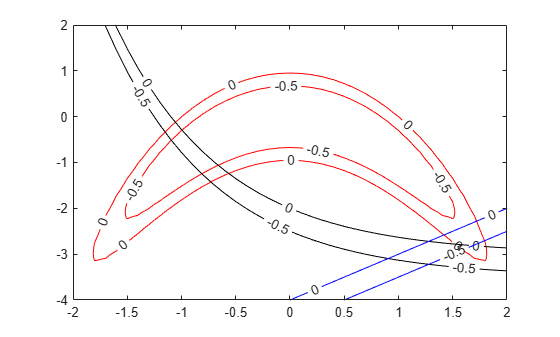There appears to be a small feasible region near $x=1.75,y=-3$. Notice that there is no point where all constraint values are below –1/2, so the feasible set is small.

### Use Problem-Based `Optimize` Live Editor Task

To find a feasible point, launch the `Optimize` Live Editor task from a Live Script by choosing Task > Optimize on the Code tab or Insert tab. Choose the problem-based task.

Set the problem variable `x` to have lower bound –5 and upper bound 5. Set the problem variable `y` to have lower bound –10 and upper bound 10. Set the initial point for `x` to 2 and for `y` to –2.

Set the Goal to Feasibilty.

Create inequalities represending the three constraints. Your task should match this picture.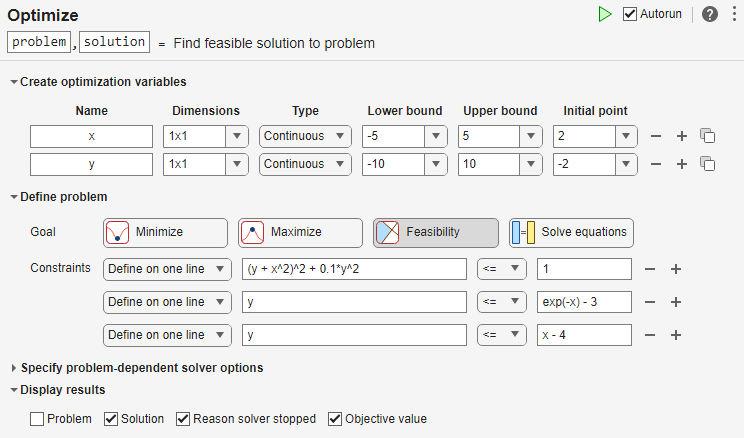Switch the task mode to Solve problem. The task chooses the `fmincon` solver, and reaches the following solution.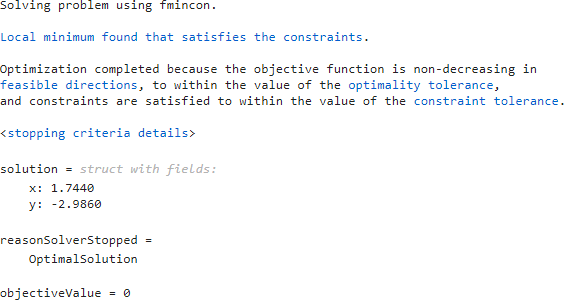### Effect of Initial Point

Starting from a different initial point can cause `fmincon` to fail to find a solution. Set the initial point for x to –2.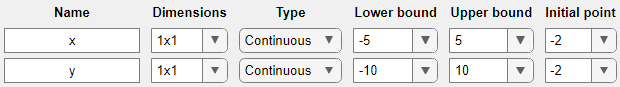This time `fmincon` fails to find a feasible solution.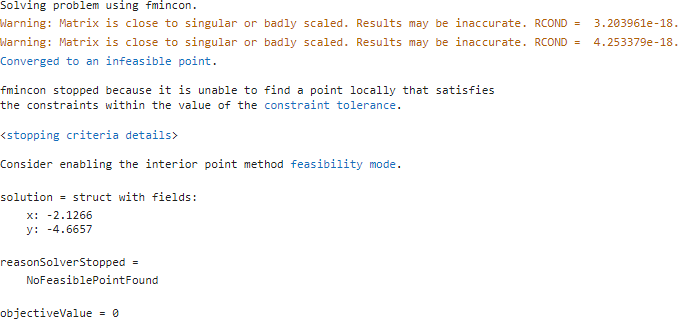### Try Different Solver

To attempt to find a solution, try a different solver. Set the solver to `ga`. To do so, specify the solver in the Specify problem-dependent solver options expander. And to monitor the solver progress, set the plot function to Max constraint violation.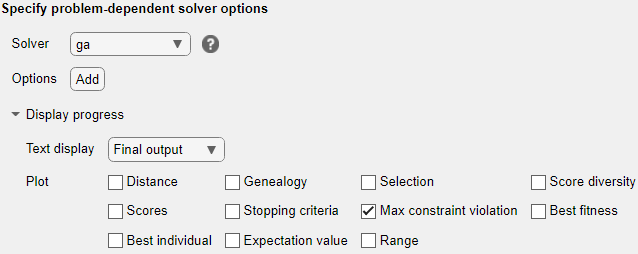`ga` finds a feasible point to within the constraint tolerance.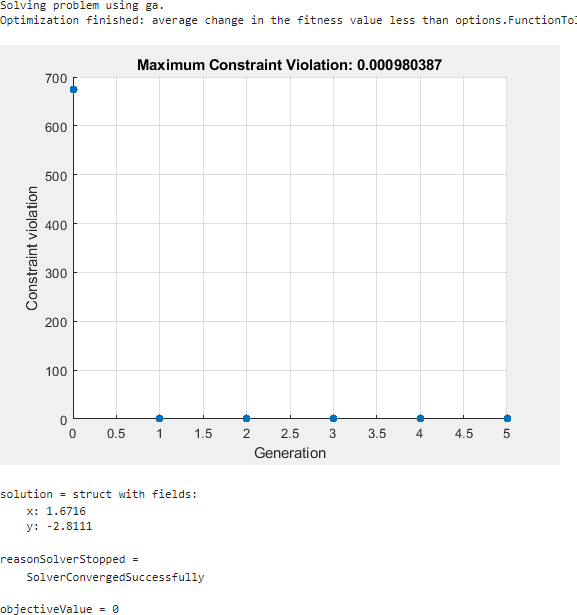`ga` finds a different solution than `fmincon`. The solution is slightly infeasible. To get a solution with lower infeasibility, you can set the constraint tolerance option to a lower value than the default. Alternatively, try a different solver.

### Try `surrogateopt`

Try using the `surrogateopt` solver. Set the plot function as Max constraint violation; this setting does not carry forward automatically from the `ga` solution.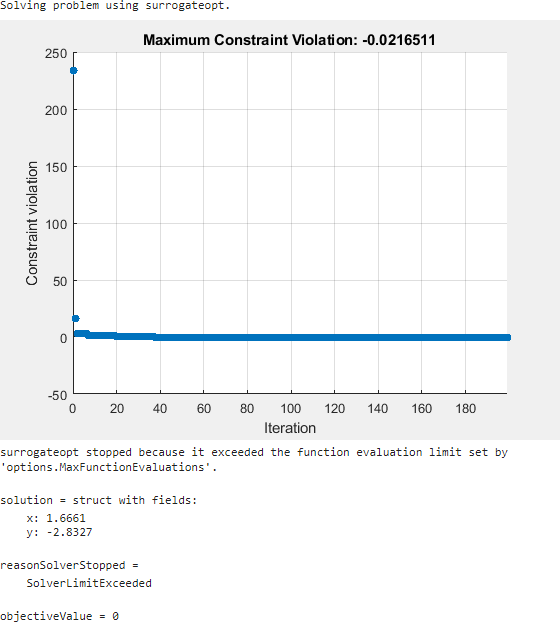`surrogateopt` reaches a feasible solution, but does not stop when it first reaches a solution. Instead, `surrogateopt` continues to iterate until it reaches its function evaluation limit. To stop the iterations earlier, specify an output function that halts the solver as soon as the maximum constraint violation reaches 1e-6 or less. Doing so causes the solver to stop much earlier. Use the `surrout` helper function, which appears at the end of this script. To specify this function, create a function handle to the function.

`outfun = @surrout;`

Specify this function handle in the Specify problem-dependent solver options > Options > Diagnostics > Output function drop-down menu.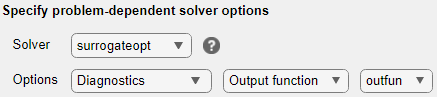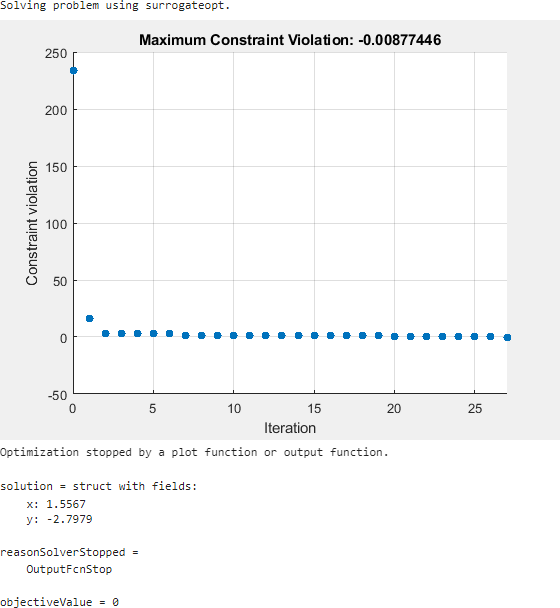This time the solver stops after about 30 function evaluations instead of 200. The solution is slightly different than the previous one, but both are feasible solutions.

### Conclusions

The problem-based `Optimize` Live Editor task helps you try using different solvers on a problem, even solvers that have different syntaxes such as `fmincon` and `surrogateopt`. The task also helps you set plot functions, and set other options.

The task appears here in its final state. Feel free to experiment using different solvers and options.

```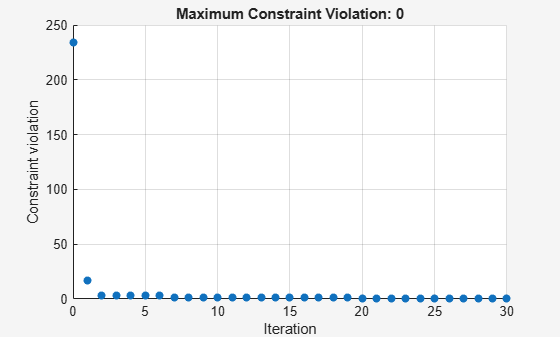```

### Helper Functions

This code creates the `plotobjconstr` helper function.

```function plotobjconstr [XX,YY] = meshgrid(-2:0.1:2,-4:0.1:2); ZZ = objconstr([XX(:),YY(:)]).Ineq; ZZ = reshape(ZZ,[size(XX),3]); h = figure; ax = gca; contour(ax,XX,YY,ZZ(:,:,1),[-1/2 0],'r','ShowText','on'); hold on contour(ax,XX,YY,ZZ(:,:,2),[-1/2 0],'k','ShowText','on'); contour(ax,XX,YY,ZZ(:,:,3),[-1/2 0],'b','ShowText','on'); hold off end```

This code creates the `objconstr` helper function.

```function f = objconstr(x) c(:,1) = (x(:,2) + x(:,1).^2).^2 + 0.1*x(:,2).^2 - 1; c(:,2) = x(:,2) - exp(-x(:,1)) + 3; c(:,3) = x(:,2) - x(:,1) + 4; f.Ineq = c; end```

This code creates the `surrout` helper function

```function stop = surrout(~,optimValues,~) stop = false; if optimValues.constrviolation <= 1e-6 % Tolerance for constraint stop = true; end end```